• 数据的多层索引以及多层索引的排序 1、多层索引的创建 对于Series对象： 示例： import pandas as pd Se=pd.Series([1,2,3,4],index=[["张三","张三","李四","李四"], ...
                                    数据的多层索引以及多层索引的排序


1、多层索引的创建
对于Series对象：
示例：
import pandas as pd
Se=pd.Series([1,2,3,4],index=[["张三","张三","李四","李四"],
["期中","期末","期中","期末"]])
print(Se)
对于DataFrame对象：
示例：
import pandas as pd
import numpy as np          #导入numpy模块用来生成数据集
list=np.random.randint(0,100,(6,3))     #生成一个6行3列的数据集
df=pd.DataFrame(data=list,index=[['张三','张三','李四','李四','王五','王五'],
['期中','期末','期中','期末','期中','期末']],
columns=["java","HTML","Python"])
print(df)

对于以上的写法创建DataFrame对象时需要写很多重复的索引值，如何才能简化索引的写法呢？
在pandas中为我们提供了一个创建多层索引的构造方法：
pd.MultiIndex.from_product()构建索引的方式

修改之后:
import pandas as pd
import numpy as np          #导入numpy模块用来生成数据集
list=np.random.randint(0,100,(6,3))     #生成一个6行3列的数据集
name=['张三','李四','王五']
xueqi=['期中','期末']
index1=pd.MultiIndex.from_product((name,xueqi))
df=pd.DataFrame(data=list,index=index1,columns=["java","HTML","Python"])
print(df)

2、多层索引的取值
对于Series对象的取值：
可以根据生成的Series对象直接获取数据，但是必须是先获取外层的再获取内层的，如下：
import pandas as pd
s = pd.Series([1,2,3,4,5,6],index=[['张三','张三','李四','李四','王五','王五'],
['期中','期末','期中','期末','期中','期末']])
print(s["张三"])
print(s[:,"期中"])

注意：只能先获取姓名之后再获取学期的数据，必须由外层索引到内层索引

对于DataFrame对象的取值：
import pandas as pd
import numpy as np
#size参数是指定生成6行3列的数组
data = np.random.randint(0,100,size=(6,3))
names = ['张三','李四','王五']
exam = ['期中','期末']
index = pd.MultiIndex.from_product([names,exam])
df = pd.DataFrame(data,index=index,columns=['Java','Web','Python'])
获取值张三期中的成绩的三种方法：
df.loc['张三','期中']
df.loc['张三'].loc['期中']
df.loc[('张三','期中')]

3、多层索引的排序
有时候，我们需要将分组或创建出来的多层索引数据，根据索引值进行排序
DataFrame按行索引排序的方法是sort_index()
sort_index()中的level参数可以指定是否按照指定的层级进行排列，第一层级索引值为0，第二层级索引值为1。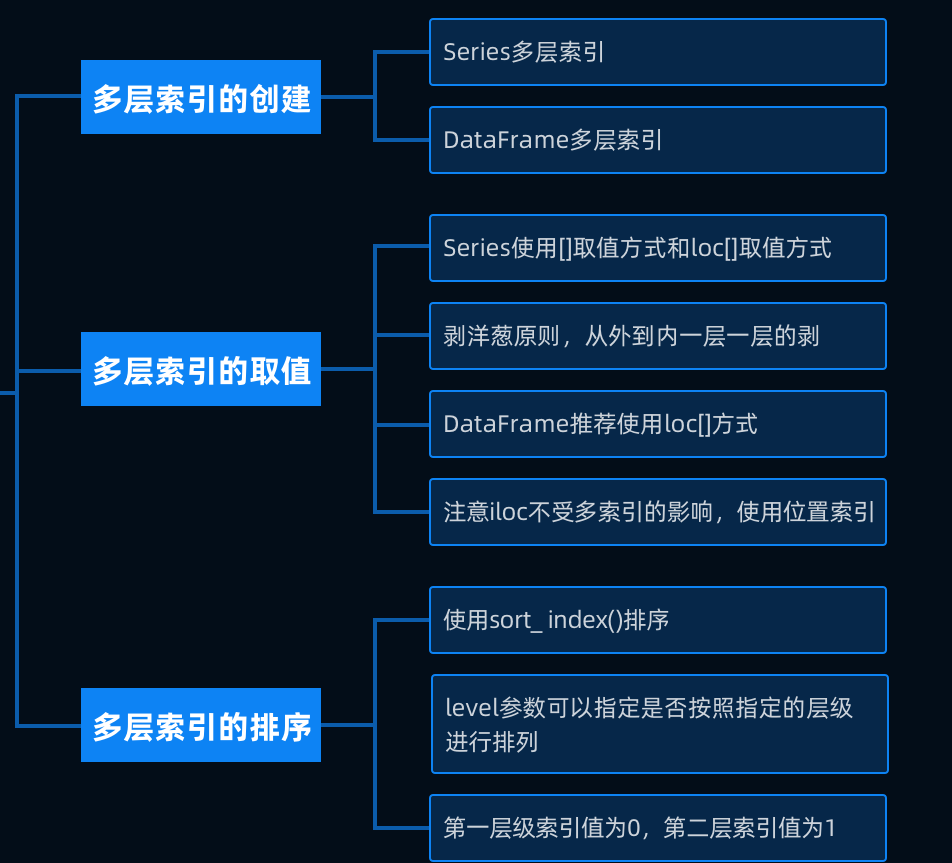展开全文• ## Carbon Data 多级索引

千次阅读 2017-08-23 11:24:57
Carbon Data 多级索引
INTRODUCTION 介绍

Apache CarbonData has Rich Multi-Level Index Support.

Apache CarbonData有丰富的多级索引支持。
DESCRIPTION 描述

Apache CarbonData uses multiple indexes at various levels to enable faster search and query processing.

Apache CarbonData使用多个级别的索引来实现更快的搜索和查询处理。

Using indexes, we can efficiently find the position of the data that is required while skipping the parts of data that are not required (need not be processed) and hence results in faster query processing.

使用索引，我们能更有效的找到数据的位置，因为我们能跳过我们不需要的数据，因此会加速查询操作。

Storing data along with index significantly accelerates query performance and reduces the I/O scans and CPU resources in case of filters in the query. CarbonData index consists of multiple levels of indices, a processing framework can leverage this index to reduce the number of tasks it needs to schedule and process. It can also do skip scan in more fine grained units (called blocklet) in task side scanning instead of scanning the whole file.

存储数据的时候使用索引，能够明显的提高查询性能，减少I/O扫描和CPU资源，因为在查询的时候，很多数据会被过滤。CarbonData的索引由多级索引组成，处理框架可以利用这个索引去减少很多需要调度和处理的任务。它也可以从一侧开始更细粒度的去扫描blocklet，而不是扫描整个文件。
To get data using indexing, the steps followed are : 为了让数据使用索引，步骤如下
File Pruning. 文件修剪Blocklet Pruning. Blocklet修剪Binary search using Inverted Index. 使用倒序索引进行二分查找
TYPES OF INDEXES 索引的类型
Index stored in file footer(enables two level of B+ tree indexing):
索引存储在file footer(两种级别的B+树索引)
Table level index: global B+ tree, efficient file level filtering.Searching for the file, using the table level index.These files will be further used, to get the row-groups(Data Blocks) using the file level index.
表级别的索引：全局的B+树，高效的文件级别过滤。在文件中检索，使用表级别的索引。这些文件将会别进一步利用，使用文件级索引去得到row-groups(Data Blocks)。图一

File level index: local B+ tree, efficient blocklet level filtering
文件级别的索引，本地的B+树，高效的blocklet级别过滤。图二

Global Multi Dimensional Keys(MDK) based B+Tree Index for all non- measure columns aids in quickly locating the row groups(Data Blocks) that contain the data matching search/filter criteria.
依据B+Tree索引的全局的Multi Dimensional Keys(MDK)帮助为测量的列去快速定位包含搜索/过滤条件匹配的数据的row groups（Data Blocks）。图三

Min-Max Index for all columns aids in quickly locating the row groups(Data Blocks) that contain the data matching search/filter criteria.
Min-Max索引帮助所有列去快速定位到包含搜索/过滤条件匹配的数据的row group(Data Blocks)图四

Column level index: inverted index used for efficient column chunk scan 列级别的索引：倒序索引，用于高效的列块扫描图五

Data Block level Inverted Index for all columns aids in quickly locating the rows that contain the data matching search/filter criteria within a row group(Data Blocks).
针对所有列的Data Block级别的倒序索引，帮助快速定位到包含数据匹配搜索和过滤的行。图六

原文地址
Multi Level Indexing - CarbonData - Apache Software Foundation
展开全文carbondata
• 本文介绍了索引文件及其基本分类，包括稠密索引文件和非稠密索引分块文件。
基本概念
索引：记录关键字值与记录的存储位置之间的对应关系；
索引文件：由基本数据与索引表两部分组成的数据文件称为索引文件；
索引表的特点： 1、索引表是由系统自动产生的； 2、索引表中表项按关键字值有序排列；
稠密索引文件
文件的基本数据中的每一个记录在索引表中都占有一项，这样的索引文件叫稠密索引文件，如下图：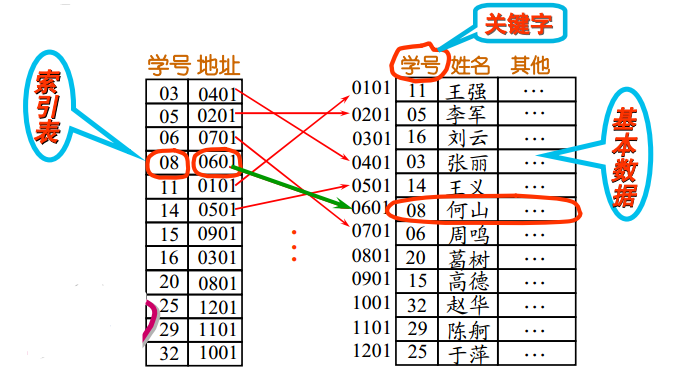由于每一项记录在索引表中都占一项，所以在稠密索引文件中查找一个记录存在与否的过程是直接查找索引表；
非稠密索引分块文件
将文件的基本数据中记录分成若干块(块与块之间记录按关键字值有序, 块内记录是否按关键字值有序无所谓)，索引表中为每一块建立一项，这样的索引文件叫非稠密索引分块文件，如下图：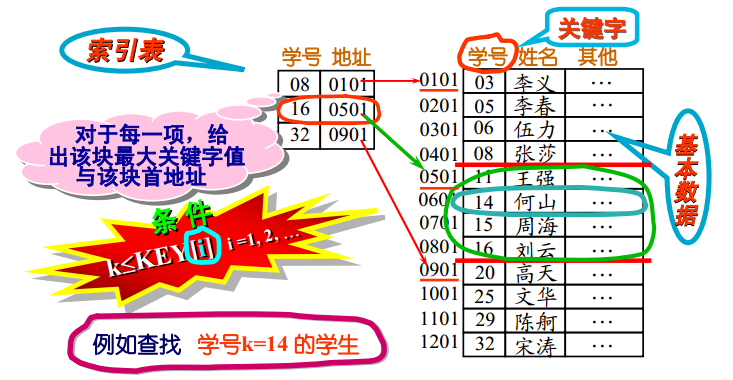在非稠密索引(分块)文件中查找一个记录存在与否的过程是： 先查找索引表（确定被查找记录所在块），然后在相应块中查找被查记录存在与否。
多级索引文件
当索引文件的索引本身非常庞大时，可以把索引分块，建立索引的索引，形成树形结构的多级索引。
展开全文索引文件 索引表
• 1. 多层索引 多层索引（MultiIndex...我们在创建Series或者DataFrame时，通过 index(columns) 参数传递多维数组，进而创建多级索引多级索引可以通过names属性设置名称，每级索引的元素个数相同。 第二种 我们 Mul...


文章目录
1. 多层索引1.1 创建方式第一种第二种
1.2. 多层索引操作1.2.1. Series 多层索引1.2.2. DataFrame 多层索引
2. 交换索引 swaplevel3. 索引排序 sort_index4. 索引堆叠 stack/unstack5. 索引设置 set_index6. 重置索引 reset_index7. 分组与聚合7.1. groupby分组7.2. 迭代7.3. 分组方式* 分组后统计：
7.4. apply7.5. 聚合

1. 多层索引
多层索引（MultiIndex），具有多个层次索引，有些类似于根据索引进行分组的形式。通过多层次索引，我们可以使用高层次索引，来操作整个索引组的数据。
1.1 创建方式
第一种
我们在创建Series或者DataFrame时，通过 index(columns) 参数传递多维数组，进而创建多级索引。多级索引可以通过names属性设置名称，每级索引的元素个数相同。
第二种
我们 MultiIndex 类的方法创建 MultiIndex 对象，然后作为Sereis或者DataFrame的index(columns)参数值。同样可以通过names参数指定多层索引的名称
from_arrays：接收一个多维数组参数，高维指定高层索引，低纬指定底层索引from_tuples：接收一个元素作为列表，每个元祖指定每个索-引->(高维索引，低纬索引)from_product：接收一个可迭代对象列表，根据可迭代对象列表中元素的笛卡尔积创建多级索引
1.2. 多层索引操作
对于多层索引，同样支持单层索引的相关操作，例如，索引元素、切片、索引数组选择元素等。我们也可以根据多级索引，按照层次逐级选择元素。多层元素优势，通过高层次索引，来操作整个索引组的数据
操作语法：
s[操作]  s.loc[操作]  s.iloc[操作]
操作可以是索引、切片、数组索引
1.2.1. Series 多层索引
loc：标签索引操作，通过多层索引，获取该索引对应的一组值  iloc：位置索引操作，获取对应位置的元素值，与是否多层索引无关  s[操作]的操作逻辑见下，不推荐使用！
对于索引(单级)，首先按照标签选择，如标签不存在，按照位置选择对于多级索引，按照标签进行选择对于切片，如果提供的是整数，按照位置选择，否则按照标签选择对于数组索引，如果数组元素都是整数，根据位置进行索引，否则根据标签索引
1.2.2. DataFrame 多层索引
loc：标签索引操作，通过多层索引，获取该索引对应的一组值  iloc：位置索引操作，获取对应位置的一行数据，与是否多层索引无关  s[操作]的操作逻辑见下，不推荐使用！
对于索引，根据标签获取相应列。如果是多层索引，获得多列对于数组索引，根据标签，获取相应列。如果是多层索引，获得多列对于切片，先按照标签索引，然后再按照位置进行索引，获取行的数据
import numpy as np
import pandas as pdSeries 创建多级索引：
# Series创建多级索引，通过index参数指定
# 单层索引，index参数指定一维数组
# s = pd.Series([1,2,3,4],index=['a','b','c','d'])
# display(s)
### 方式01 ###
# 1.多级 索引，index参数值指定多维数组
# s = pd.Series([1,2,3,4],index=[['湖南','湖南','北京','北京'],['长沙','衡阳','海淀','昌平']])
# display(s)
# # 多级索引，为每一层索引指定名称
# s.index.names=['省份','城市']
# display(s)

### 方式02 ###
# 2.Series创建多级索引，通过创建MultiIndex对象，设置给index参数
# from_arrays，通过列表的方式创建：[[第1级索引],[第2级索引],.....[第n级索引]]
# m = pd.MultiIndex.from_arrays([['湖南','湖南','北京','北京'],['长沙','衡阳','海淀','昌平']])
# s = pd.Series([1,2,3,4],index=m)
# display(s)

### 方式03 ###
# 3.from_tuples：通过元祖构成列表，[(第1级索引元素,第2级索引元素),(第1级索引元素,第2级索引元素)....]
# m = pd.MultiIndex.from_tuples([('湖南','长沙'),('湖南','衡阳'),('北京','海淀'),('北京','昌平')])
# s = pd.Series([1,2,3,4],index=m)
# display(s)
### 方式04 ###
# 4.from_product：通过笛卡尔积方式创建MultiIndex对象设置给index参数，但是结果不合理！
m = pd.MultiIndex.from_product([['湖南','北京'],['长沙','衡阳','海淀','昌平']])
s = pd.Series([1,2,3,4,5,6,7,8],index=m)
display(s)



DataFrame 行多级索引：
# DataFrame行多级索引
# 它和Series多级索引创建没有区别，方式1：index参数多维数组。
# 方式2：通过MultiIndex类的方法(from_arrays from_tuples from_product)创建MultiIndex对象，设置index。

### 方式01 ###
# df = pd.DataFrame(np.arange(1,9).reshape(4,2),index=[['湖南','湖南','北京','北京'],['长沙','衡阳','海淀','昌平']])
# display(df)

### 方式02 ###
# from_arrays
# m = pd.MultiIndex.from_arrays([['湖南','湖南','北京','北京'],['长沙','衡阳','海淀','昌平']])
# df = pd.DataFrame(np.arange(1,9).reshape(4,2),index=m)
# display(df)

### 方式03 ###
# from_tuples
# m = pd.MultiIndex.from_tuples([('湖南','长沙'),('湖南','衡阳'),('北京','海淀'),('北京','昌平')])
# df = pd.DataFrame(np.arange(1,9).reshape(4,2),index=m)
# display(df)

### 方式04 ###
# from_product
m = pd.MultiIndex.from_product([['湖南','北京'],['长沙','衡阳','海淀','昌平']])
df = pd.DataFrame(np.arange(1,17).reshape(8,2),index=m)
display(df)



创建 DataFrame 列多级索引：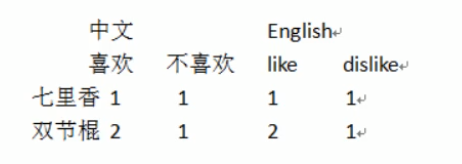# DataFrame创建列多层索引和行创建多层索引类似，区别在创建DataFrame设置给参数columns
df = pd.DataFrame(np.array([[1,1,1,1],[2,1,2,1]]),index=['七里香','双节棍'],columns=[['中文','中文','English','English'],['喜欢','不喜欢','like','dislike']])
display(df)



Series 多层索引操作：
# Sereis多层索引操作
# 1.loc标签操作
s = pd.Series([1,2,3,4],index=[['湖南','湖南','北京','北京'],['长沙','衡阳','海淀','昌平']])
display(s)
# 直接使用外层索引访问,可以获得外层索引对应的一组值
# display(s.loc['湖南'])

# 多层索引访问中，不支持直接使用内层索引访问
# 因为多层索引访问中，首先从外层索引找，外层没有‘长沙’标签，报错！
# display(s.loc['长沙'])
# 使用外层+内存索引逐层访问
# display(s.loc['湖南','长沙'])

# 2.iloc位置操作
# 根据位置访问，与是否存在多层索引没有关系的
# display(s.iloc)

# 3.切片操作
# 根据【标签】来切片，注意切片的标签需要排好序（字典顺序），否则报错！（详见下段代码）
# s = s.sort_index()
# display(s)
# display(s.loc['北京':'湖南'])
# 根据【位置】切片
# display(s.iloc[0:2])

# 4.数组索引,也许要对索引进行进行排序
s = s.sort_index()
display(s.loc[['北京','湖南']])


注意：标签来切片，注意切片的标签需要排好序（字典顺序），否则报错。使用 sort_index 方法对索引进行排序（字典顺序），再切片！
# 标签来切片，注意切片的标签需要排好序（字典顺序），否则报错。使用sort_index方法对索引进行排序（字典顺序），再切片
s= pd.Series([1,2,3,4],index=[['b','a','a','b'],['长沙','衡阳','海淀','昌平']])
display(s)
s  = s.sort_index()
display(s)
display(s.loc['b':'a'])



DataFrame 多层索引操作：m =pd.MultiIndex.from_product([['湖南','北京'],['2017','2018']])
df = pd.DataFrame(np.array([,,,]),index=m)
display(df)

# 1.loc 标签的访问
# 直接使用外层索引访问
# display(df.loc['湖南'])
# 不支持直接使用内层索引访问
# display(df.loc['2017'])
# 外层+内层逐层访问，得到一行数据
# display(df.loc['湖南','2017'])
# display(df.loc[('湖南','2017')])

# 2.iloc位置访问,是否存在多层索引没有关系的
# display(df.iloc)

# 3.切片操作
# 标签的切片
# 先对索引进行排序
# df = df.sort_index()
# display(df)
# # display(df.loc['北京':'湖南'])
# # 混合操作,设置axis=0，否则2017当做列索引名称。但是Series不需要！（见下方代码）
# display(df.loc(axis=0)[:,'2017'])

# 4.位置切片
# display(df.iloc[0:2])

# 5.数组索引,也需要索引有序
df = df.sort_index()
display(df)
display(df.loc[['北京','湖南']])


注意：混合操作,设置axis=0，否则2017当做列索引名称。但是Series不需要！
# Series 不需要设置 axis=0
m =pd.MultiIndex.from_product([['湖南','北京'],['2017','2018']])
s = pd.Series(np.array([8000,8100,1200,1150]),index=m)
display(s.loc[:,'2017'])



2. 交换索引 swaplevel
我们可以调用DataFrame对象的swaplevel方法交换两个层级索引。该方法默认对倒数第2层和倒数第1层进行交换。
我们可以指定交换层级，层次从0开始，由外向内递增，也可以指定负值，负值表示倒数第n层。
我们可以通过层级索引的名称进行交换。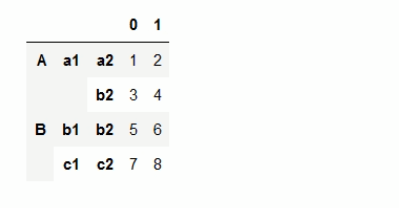df = pd.DataFrame(np.arange(1,9).reshape(4,2),index=[['A','A','B','B'],['a1','a1','b1','c1'],['a2','b2','b2','c2']])
display(df)

### 方式01 ###
# 默认交换最里面2个层级索引
# display(df.swaplevel())
# 索引的层级从外到内，第0层，第1层，第2层
display(df.swaplevel(0,2))  # 第0层和第2层叫唤
# 索引的层级可以为负值，-1，-2，-3，-1最内层
display(df.swaplevel(-3,-1))

### 方式02 ###
# 根据层级索引名称交换
df.index.names=['layer0','layer1','layer2']
display(df.swaplevel('layer0','layer2'))



3. 索引排序 sort_index
我们可以使用 sort_index 对索引进行排序处理。
参数：
level：指定根据哪一层排序，默认最外层。level值可以是数值，索引名或者二者构成的列表。inplace：是否就地修改，默认为False。
# 对行进行多层索引排序：
df = pd.DataFrame(np.arange(1,9).reshape(4,2),index=[['B','A','B','A'],['a1','a1','b1','c1'],['a2','b2','b2','c2']])
display(df)

# 索引排序，默认对最外层的索引排序(字典升序)
# display(df.sort_index())
# 自定义对哪个层级的索引排序
# df.sort_index(level=1)
df.sort_index(level=1,ascending=False)

# inplace参数：
df.sort_index(inplace=True)
display(df)



对列进行多层索引排序：
# 对列进行多层索引排序：
df = pd.DataFrame(np.array([[1,1,1,1],[2,1,2,1]]),index=['七里香','双节棍'],columns=[['Chinese','Chinese','English','English'],['喜欢','不喜欢','like','dislike']])
display(df)
display(df.sort_index(axis=1,ascending=False))



4. 索引堆叠 stack/unstack
通过 DataFrame 对象的stack方法，可以进行索引堆叠，即将指定成绩的列转换成行。
level参数：指定转换的层级，默认为- 1。  通过 DataFrame 对象的unstack方法，可以取消索引堆叠，即将指定层级的行转换成列。
level参数：指定转换的层级，默认为 -1。  fill_value参数：指定填充值，默认为NaN。
# 对索引进行堆叠操作，会将列索引堆加在行最内层索引上。
# 当对索引进行取消堆叠的操作，将行索引堆加列的最内层索引上
# 索引堆叠使得访问数据更加灵活

m = pd.MultiIndex.from_arrays([['湖南','湖南','北京','北京'],['东部','西部','东部','西部']])
df = pd.DataFrame(np.arange(1,9).reshape(4,2),index=m,columns=['人口','GDP'])
display(df)

# 当对索引进行取消堆叠的操作，将行索引堆加列的最内层索引上
df = df.unstack()
display(df)
# # 访问人口是东部的数据
display(df['人口','东部'])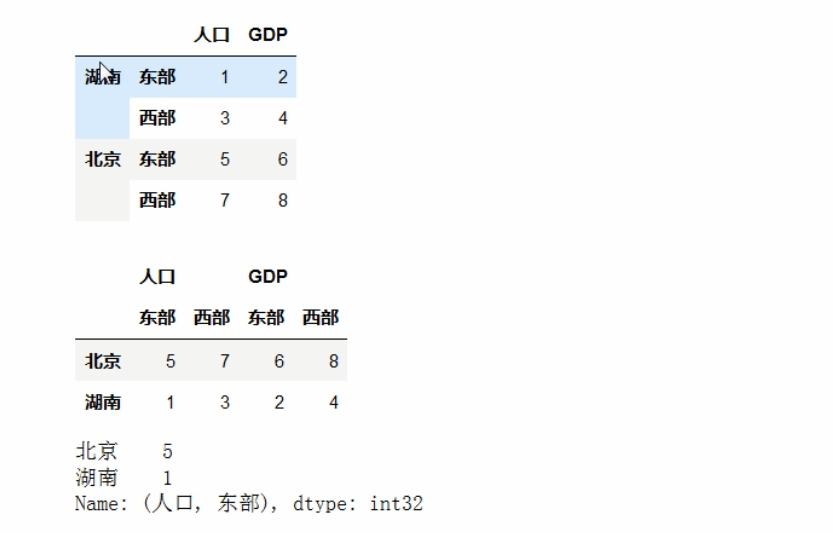df = pd.DataFrame(np.arange(1,9).reshape(4,2),index=[['B','A','B','A'],['a1','a1','b1','c1'],['a2','b2','b2','c2']])
df.index.names=['layer0','layer1','layer2']
df.sort_index(inplace=True)
display(df)

# 取消堆叠操作，可能会产生NaN，避免空值，填充数据使用fill_value
# df = df.unstack(fill_value=0)
# display(df)

# level参数自定义层级，默认是行最内层索引
# df = df.unstack(level=1,fill_value=0)
# display(df)

# 除了自定义层级数值，还可以指定索引的名称
df = df.unstack(level='layer0',fill_value=0)
display(df)



列索引堆叠：
# 索引的堆叠操作 对索引进行堆叠操作，会将列索引堆加在行最内层索引上
df = pd.DataFrame(np.array([[1,1,1,1],[2,1,2,1]]),index=['七里香','双节棍'],columns=[['中文','中文','English','English'],['喜欢','不喜欢','like','dislike']])
display(df)
# 七里香歌曲中中文信息
df = df.stack(0)
display(df)
display(df.loc['七里香','中文'])


5. 索引设置 set_index
在DataFrame中，如果我们需要将现有的某一或者多列作为行索引，可以调用set_index方法实现。
参数：
drop：是否丢弃作为新索引列，默认为Trueappend：是否以追加方式设置索引，默认为Falseinplace：是否就地修改，默认为False
df = pd.DataFrame({'stuno':[1,2,3],'name':['zs','ls','ww'],'age':[20,21,2]})
display(df)

# 1.set_index设置索引，设置参数指定列，充当索引
# display(df.set_index('stuno'))

# 2.设置层级行索引
# display(df.set_index(['stuno','name']))

# 3.默认情况下，充当行索引的列数据丢弃，设置drop=false保留列数据
# display(df.set_index('stuno',drop=False))

# 4.append用来设置是否以追加的方式设置索引，默认False(取代之前的索引)
df.set_index('stuno',inplace=True)
display(df)
df.set_index('name',inplace=True,append=True)
display(df)



6. 重置索引 reset_index
调用DataFrame的reset_index重置索引（将行索引取消），与set_index正相反。
参数：
level：重置索引层级，默认重置所有层级的索引。如果重置所有索引，将会创建默认整数序列索引。drop：是否丢弃重置索引列，默认为False。inplace：是否就地修改，默认为False。
df = pd.DataFrame({'stuno':[1,2,3],'name':['zs','ls','ww'],'age':[20,21,2]})
# display(df)
# 设置层级行索引
df.set_index(['stuno','name'],inplace=True)
display(df)

# 1.重置索引，默认重置所有层级的行索引，重新生成整数序列为行索引
# display(df.reset_index())

# 2.level指定重置索引的层级，将重置的行索引充当回新的列
# display(df.reset_index(1))

# 3.重置索引后，默认将重置的行索引充当回新的列，如果不想将重置的行索引充当回新的列，指定drop=True
display(df.reset_index(1,drop=True))



7. 分组与聚合
分组与聚合操作与数据库分组和聚合类似
7.1. groupby分组
我们可以通过groupby方法对Series或DataFrame对象实现分组操作。该方法返回一个分组对象。 分组对象属性和方法：
groups属性:返回一个字典类型对象，包含分组信息size方法:返回每组记录数量describe方法:分组查看统计信息
7.2. 迭代
使用for循环对分组对象进行迭代。迭代每次返回一个元祖（tuple）；第1个元素为分组的key，第2个为改组对应的数据（value）。
df = pd.DataFrame({'部门':['研发','财务','研发','财务'],'项目组':['一组','二组','二组','一组'],
'姓名':['张三','李四','王五','赵六'],'年龄':[20,22,23,24],'利润':[5,10,10,25]})
display(df)

# 根据单个列索引分组，返回分组对象
group = df.groupby('部门')
# 分组对象不像列表，可以将分组后内容直接输出，其类型是DataFrameGroupBy
display(group)

# 分组对象是一个可迭代的对象，通过for循环来查看分组信息
# for item in group:
#     display(type(item))  # 返回的是tuple

# 对分组对象迭代返回的元组对象(key,value)
for k,v in group:
display(k,v)

# 查看分组对象属性和方法
# groups属性得到分组信息字典 key：分组数据的索引
display(group.groups)
# 得到每组记录条数
display(group.size())
display(group.describe())


7.3. 分组方式
使用groupby进行分组时，分组参数可以是如下形式：
索引名：根据该索引进行分组。  函数：函数需要有一个参数，用来接收行索引值。函数还需要具有返回值，用来指定组。  索引名构成的数组：根据数组中多个索引进行分组。  字典或者Series：key指定索引，value指定分组依据，value值相等的分为一组。
df = pd.DataFrame({'部门':['研发','财务','研发','财务'],'项目组':['一组','二组','二组','一组'],
'姓名':['张三','李四','王五','赵六'],'年龄':[20,22,23,24],'利润':[5,10,10,25]})
display(df)

# 根据单个列说明分组，返回分组对象
# group = df.groupby('部门')
# for k,v in group:
#     display(k,v)

### 方式01 ###
# 1.多个索引列分组
# group = df.groupby(['部门','项目组'])
# for k,v in group:
#     display(k,v)

### 方式02 ###
# 2.根据函数分组
# df = df.set_index('利润')
# display(df)
# # 函数需要有一个参数，用来接收行索引值。函数还需要具有返回值，用来指定组
# def group_handle(index):
#     if index<10:
#         return 0
#     return 1
# # 需求：将利润<10和利润>=10的人员分组
# group = df.groupby(group_handle)
# for k,v in group:
#     display(k,v)

### 方式03 ###
# 3.字典
# 根据行索引分组
# group = df.groupby({0:0,1:0,2:1,3:1})
# for k,v in group:
#     display(k,v)

# 4.根据列索引值分组
group = df.groupby({'姓名':0,'利润':0,'部门':1,'项目组':1},axis=1)
for k,v in group:
display(k,v)



* 分组后统计：
对分组数据统计(聚合)：利润、年龄、数值型进行统计；姓名、项目组是字符串，不会统计；部门属于分组字段，不参与统计。对 DataFrame：所有数值型数据相加，字符串做拼接；设置DataFrame只对数值型数据统计，指定参数numeric_only=True。对分组数据中指定列数据进行统计。
df = pd.DataFrame({'部门':['研发','财务','研发','财务'],'项目组':['一组','二组','二组','一组'],
'姓名':['张三','李四','王五','赵六'],'年龄':[20,22,23,24],'利润':[5,10,10,25]})
display(df)

group= df.groupby('部门')
for k,v in group:
display(k,v)

# 1.对分组数据统计(聚合)
# 利润、年龄、数值型进行统计；姓名、项目组是字符串，不会统计；部门属于分组字段，不参与统计
display(group.sum())

# 2.对比DataFrame
# 所有数值型数据相加，字符串做拼接
display(df.sum())
# 设置DataFrame只对数值型数据统计，指定参数numeric_only=True
display(df.sum(numeric_only=True))

# 3.对分组数据中指定列数据进行统计
display(group['利润'].sum())



7.4. apply
对分组对象，可以调用apply函数，该函数接收每个组的数据，返回操作之后的结果。apply最后将每个组的操作结果进行合并（concat）。
df = pd.DataFrame({'部门':['研发','财务','研发','财务'],'项目组':['一组','二组','二组','一组'],
'姓名':['张三','李四','王五','赵六'],'年龄':[20,22,23,24],'利润':[5,10,10,25]})
display(df)

group= df.groupby('部门')
for k,v in group:
display(k,v)

# 对分组对象进行apply，apply接收函数实现对分组数据操作
# 函数具有一个参数，依次接收分组数据，返回每一个分组处理的结果，
# 注意点，apply对于第一个分组数据会调用2次，但是不会影响操作结果
# group.apply(lambda x:display(x,type(x)))

# 对每组数据进行求和统计
display(group.apply(lambda x:x.sum(numeric_only=True)))



7.5. 聚合
可以在分组对象进行聚合（多个值变成一个值）。例如，mean()，sum()等。
除此之外，我们可调用agg方法，实现自定义聚合方式。函数接收一行或者一列数据，返回该行或者列聚合后的结果。  agg方法实现对DataFrame、分组对象的聚合，可以传入4种类型参数，字符串、列表、字典以及函数。
示例：
agg 方法实现DataFrame对象的聚合：
				### agg方法实现DataFrame对象的聚合 ###
df = pd.DataFrame({'部门':['研发','财务','研发','财务'],'项目组':['一组','二组','二组','一组'],
'姓名':['张三','李四','王五','赵六'],'年龄':[20,22,23,24],'利润':[5,10,10,25]})
# display(df)

# 使用mean sum这些统计方法得到聚合信息
# display(df.mean(numeric_only=True),df.sum(numeric_only=True))

### agg方法实现对DataFrame、分组对象的聚合，可以传入4种类型参数，字符串、列表、字典以及函数 ###
# 1.字符串
# display(df.agg('mean',numeric_only=True),df.agg('sum',numeric_only=True))

# 2.多个字符串构成的列表
# 优点：可以将mean和sum这两个聚合信息合并起来展示
# display(df.agg(['mean','sum']))
# display(df.agg(['mean','sum'])，numeric_only=True)

# 3.字典：{k1:v1,k2:v2}；k:指定索引名称，value:指定统计函数名
# 针对不同的列，提供不同统计聚合方法
display(df.agg({'利润':['mean','sum'],'年龄':['max','min']}))

# 4.函数
# 自定义聚合方式，函数具有一个参数，用来DateFrame传递过每一列(行)，返回操作后结果
# display(df.agg(lambda x:display(x,type(x))))
display(df.agg(lambda x:x.mean() if x.name=='利润' else None))


agg 方法实现对分组对象的聚合：
				### agg方法实现对分组对象的聚合 ###
df = pd.DataFrame({'部门':['研发','财务','研发','财务'],'项目组':['一组','二组','二组','一组'],
'姓名':['张三','李四','王五','赵六'],'年龄':[20,22,23,24],'利润':[5,10,10,25]})
# display(df)

group= df.groupby('部门')
for k,v in group:
display(k,v)

# 1.字符串
# display(group.agg('mean'))
# 2.列表
# display(group.agg(['mean','sum']))
# 3.字典
# display(group.agg({'利润':['mean','sum'],'年龄':['max','min']}))
# 4.函数
# 函数的参数是分组后得到的DataFrame的每列的数据
# display(group.agg(lambda x:display(x,type(x))))
display(group.agg(lambda x:x.mean() if x.name=='利润' else None))

展开全文• 因为BST树的插入容易导致树不平衡，树的深度太大将导致从根节点到叶节点的路径经过的磁盘太多。基于解决这两个问题的基础上，提出2-3树结构 1.2-3树 1.形状的定义： 1）一个结点包含一个或者两个关键码 2）每个...结构
• B-树 1 .B-树定义 B-树是一种平衡的多路查找树，它在文件系统中很有用。 定义：一棵m 阶的B-树，或者为空树，或为满足下列特性的m...⑷所有的非终端结点中包含以下信息数据：  （n，A0，K1，A1，K2，…，Kn，An）存储引擎
• ## 操作系统-UNIX三级索引技术

千次阅读 多人点赞 2019-11-03 10:35:29
UNIX三级索引技术 目标：学透软考的一道题目 ...存储空间会被划分成n个物理，在索引文件中，一个文件会被放入不同的物理，这时需要索引表指出一个文件分别被拆分存在哪个，所以索引表里存的是文...操作系统 Unix
• ## 计算机组成原理

万次阅读 多人点赞 2019-06-02 14:13:55
数据块的大小是16个字节，Cache的大小是64K字节。采用两路组相联映射，给内存地址为F0010H和CABBEH两个地址对应的标记、组号和字号。 解:按字节寻址,数据块为16个字节 字号:16B/1B=2 4,4位 采用两路组相联映射(每...计算机组成原理
• ## 索引-数据测试

千次阅读 2017-12-19 17:27:54
插入数据：使用PL/SQL工具，开启一个command window(命令窗口)，通过exec 包名.存储过程名，来插入数据，打开 set timing on; 执行存储过程后会有执行时间。 查询数据：在本电脑上使用Navicat Premium工具，直接执行...
• 设文件索引结点中有7个地址项，其中4个地址项是直接地址索引，2个地址项是一级间接地址索引，1个地址项是二级间接地址索引，每个地址项大小为4B，若磁盘索引块和磁盘数据块大小均为256B，则可表示的单个文件最大长度...操作系统 经验分享
• 文章目录文件的物理结构知识总览文件分配方式--索引分配索引分配（总结）超级超级重要考点：知识点回顾与重要考点文件存储空间管理知识总览存储空间的划分与初始化存储空间管理--空闲表法存储空间管理--空闲链表法...
• 5}不是Python的有效数据类型 range (x,y) for i in range ( 0 , 2 ) : print ( i ) #输出结果为：0 1 程序的三种基本结构： 顺序 结构， 循环 结构和 分支 结构 循环 是程序根据条件判断结果...
• 【操作系统/OS笔记20】打开文件、文件数据块分配、空闲空间管理、多磁盘管理（RAID）、磁盘调度算法概述 本次笔记内容： ...索引分配方法三扩展：大文件多级索引块空闲空间管理多磁盘管理-RAID（Red操作系统
• 数据库系统中文件索引的工作方式类似书的索引 如查找书中某个特定主题，可在书的索引查找主题，找到出现的页，然后读这些页，寻找我们需要的信息 数据库系统中的索引与图书馆中书的索引所起作用一样， 如为依据给定...
• innodb表默认创建为聚簇索引，与oracle的索引组织表（iot）类似，页节点不存储rowid，而是存储数据本身，聚簇索引不是一种单独的索引类型，而是一种数据存储方式，也就是索引组织表 二级索引的叶节点存放了key键值...innodb myISAM
• 数据文件的组织 字段的表示：每个字段均被SQL定义成具体的数据类型，表示成一定长度的字节序列。 记录的表示： 定长记录：它所包含的所有字段均为定长。 变长记录：允许它包含变长字段，有多种表示方法。...
• Pj—指针，指向索引块或数据块数据块中记录的指针 一个节点就是一个索引块，包含有n-1个索引项(<索引字段值Ki, 指针Pi>)+1个指针(Pn); 示例：存储块= 4096 Byte，整数型索引 字段值= 4 Byte、 指针= 8 Byte...
• 索引就是把一个关键字与它...索引按照结构可以分为线性索引，树形索引和多级索引。我们这里就只介绍线性索引技术。 所谓线性索引就是将索引项集合组织为线性结构，也成为索引表。分为：稠密索引，分块索引和倒排索引。
• 当系统需要分配一个指定大小为r的内存时，需要计算出相应的两级位图的值，其公式如下所示： 为了有一个直观的结果，我们假设SLI=4，即第二级索引将一级的内存大小范围划分为2∧SLI=16，则一级索引f=8，二级索引s...算法 嵌入式 内存管理
• Hive索引可以建立在表中的某些列上，以提升一些操作的效率，例如减少MapReduce任务中需要读取的数据块的数量。 在可以预见到分区数据非常庞大的情况下，索引常常是优于分区的。 虽然Hive并不像事物数据库那样针对...hive
• 每个inode存储文件系统对象的数据的属性和磁盘位置（多个）。的文件系统对象的属性可包括操纵元数据（例如，修改，JVSANTEN的访问，修改时间），以及雇主和权限数据（例如组ID，用户ID，权限）。 目录是分配给...
• 我们常说的“数据库”，比如“MySQL”、“Oracle”等，其实严格来说是DBMS(Database Management System)，数据库只是一个存储数据数据的仓库，而DBMS做的事是让我们能够操作数据库，比如解析SQL、DML等，都是DBMS...
• 索引条目26B，一个索引块最多可以存放307个索引条目，根节点最多指向307个分支节点，分支节点最多指向307个叶子节点 索引高度为0，索引段最大8KB 索引高度为 1 ，索引段最大(1+307)*8KB= 2.4M 索引高度为 2 ，索引...
• 概要引入基本概念线性索引之稠密索引线性索引之分块索引线性索引之倒排索引 引入 &amp;nbsp;&amp;nbsp;&amp;nbsp;&amp;nbsp;&amp;nbsp;&amp;nbsp;&amp;nbsp;折半查找、插值查找以及...程序员内功
• ## C++索引

千次阅读 2018-07-20 09:05:52
结构采用多级索引 主索引 柱面索引 磁道索引 在采用基于 B+ 树的 VSAM ( Virtual Storage Access Method ) 技术之前，IBM 公司曾经 广泛地采用 ISAM 技术 倒排索引(Inverted Index) 基于属性的倒排 ...
• 01 HFile索引结构解析HFile中索引结构根据索引层级的不同分为两种：single-level和mutil-level，前者表示单层索引，后者表示多级索引，一般为两级或三级。HFile V1版本中只有single-level一种索引结构，V2版本中...HFile HBase
• 文章目录索引：为加快查找速度而设计的一种数据结构三种线性索引稠密索引索引项一定有序优缺点分块索引数据集分块有序，每一对应一个索引内无序，间有序索引项结构间有序查找（快）+内顺序查找（慢...
• 索引能够提高数据库的查询效率，没有索引的话，查询会进行全表扫描（scan every document in a collection）,严重降低了查询效率。默认情况下，Mongo在一个集合（collection）创建时，自动地对集合的_id创建了唯一...
• 【2010 考研 408 统考真题】设文件索引结点中有 7 个地址项，其中 4 个地址项是直接地址索引，2 个地址项是一级间接地址索引，1 个地址项是二级间接地址索引，每个地址项大小为 4B，若磁盘索引块和磁盘数据块大小均...
• 2、数据量少的字段不需要加索引 3、如果where条件中是OR关系，加索引不起作用 4、符合最左原则 联合索引又叫复合索引。对于复合索引:Mysql从左到右的使用索引中的字段，一个查询可以只使用索引中的一部......